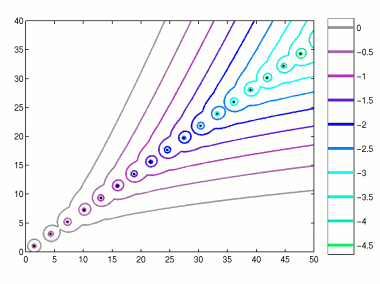Home | Introduction | Software | Examples | History | People | Bibliography | Applications | Theorems

## Pseudospectra of a Spectral Approximation of an Anharmonic OscillatorThis figure illustrates pseudospectra of an anharmonic oscillator, -uxx + cx2u, for c=1+3i. The figure above was obtained from a spectral approximation of dimension N=69. Since this discretization includes numerous spurious eigenvalues, the matrix was then projected onto an appropriate invariant subspace to accelerate computation of the pseudospectra. This example was first studied by Davies [Dav99a], [Dav00b].

Use the following MATLAB code compute a similar image using EigTool.

```  N = 70;
[D,x] = cheb(N); % cheb.m from Trefethen's "Spectral Methods in MATLAB"
x = x(2:N);
L = 6; x = L*x; D = D/L;                   % rescale to [-L,L]
A = -D^2; A = A(2:N,2:N) + (1+3i)*diag(x.^2);
opts.npts=50;
opts.ax = [0 50 0 40];
opts.levels = -10:.5:0;
opts.proj_lev = .7;
eigtool(A,opts)
```
See also program `p24.m` in L. N. Trefethen, Spectral Methods in MATLAB, SIAM, Philadelphia, 2000.